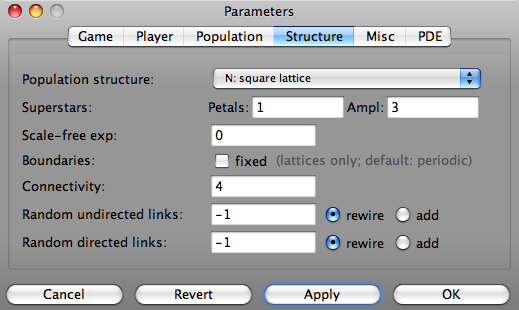# Parameters/Structure##### Panel to set the population structure

Populations have different characteristic structures determined by the type of interactions of one player with other members of the populations.

Structure
mean-field/well-mixed populations
Well mixed population without any structures, i.e. groups or pairwise encounters are formed randomly. This is often called the mean-field approximation.
complete graph
x
linear lattice
The players are arranged on a straight line - that is actually on a ring in order to reduce finite size and boundary effects - and interact with equal numbers of neighbors to their left and right.
square lattice
All players are arranged on a rectangular lattice with periodic boundary conditions. The neighborhood size may be four (von Neumann-) or eight (Moore neighborhood).
honeycomb lattice
The players are arranged on a hexagonal or honeycomb lattice interacting with their six nearest neighbors.
triangular lattice
The players are arranged on a triangular lattice interacting with their three nearest neighbors.
star
x
super-star
x
wheel
x
random regular graph
x
random graph
Randomly drawn bonds/connections between players. The neighborhood size determines the average number of bonds (average connectivity) of one player, i.e. the players interact with different numbers of other individuals.
random graph (directed)
x
scale-free graph
x
scale-free graph (Klemm)
x
scale-free graph (Barabasi & Albert)
x
linear asymmetric
x
Superstars
petals
x.
amplification
x.
Scale-free exponent
x.
Boundaries
x.
Connectivity
x.